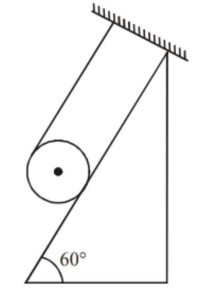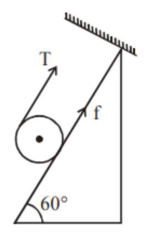# A solid cylinder of mass m is wrapped with an inextensible light string and,Question:

A solid cylinder of mass $m$ is wrapped with an inextensible light string and, is placed on a rough inclined plane as shown in the figure. The frictional force acting between the cylinder and the inclined plane is :[The coefficient of static friction, $\mu_{\mathrm{s}}$, is $\left.0.4\right]$

1. $\frac{7}{2} \mathrm{mg}$

2. $5 \mathrm{mg}$

3. $\frac{m g}{5}$

4. 0

Correct Option: , 3

Solution:

(3)Let's take solid cylinder is in equilibrium

$\mathrm{T}+\mathrm{f}=\mathrm{mg} \sin 60 \ldots$ (i)

$\mathrm{TR}-\mathrm{fR}=0 \ldots$ (ii)

Solving we get

$\mathrm{T}=\mathrm{f}_{\mathrm{req}}=\frac{\mathrm{mg} \sin \theta}{2}$

But limiting friction $<$ required friction

$\mu \mathrm{mg} \cos 60^{\circ}<\frac{\mathrm{mg} \sin 60^{\circ}}{2}$

$\therefore$ Hence cylinder will not remain in equilibrium Hence $f=$ kinetic

$=\mu_{\mathrm{k}} \mathrm{N}$

$=\mu_{\mathrm{k}} \mathrm{mg} \cos 60^{\circ}$

$=\frac{\mathrm{mg}}{5}$